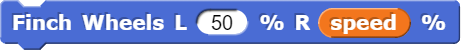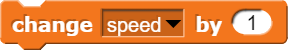# Robots @ SIT

Get hold of one of the available Robots at SIT Library and start your robotics journey today!

## Finch - Drawing Images

Required Knowledge and Parts:

• Loops, Variables, Recursion
• Movements motor, obstacle sensors

Application:

The marker holder on the Finch allows for the drawing of images. As robots will only move according to what it is told to do, they can draw very accurate pictures that humans have a hard time doing. Examples include spirals, Koch Fractals and even drawing people.

## Drawing shapes - Basic

Insert a marker into the Finch pen mount slot and use it to draw shapes!

Write a program to draw shapes. Start by drawing a square, then try to draw an equilateral triangle (a triangle with 3 equal sides). Consider how much you need to turn the Finch to draw other regular polygons, such as a hexagon or octagon. Remember that you can use a loop to repeat actions!

Once you have succeeded at regular polygons, move on to shapes where the sides are not all equal – for example, a rectangle or a trapezoid.

Snap!:

1. Use the Finch Move block to move the Finch forward a certain distance.

2. Use the Finch Turn block to turn the Finch 90 degrees to the right.

3. Repeat steps 1 and 2 such that the Finch will return to its original spot.

4. To use a repeat loop, put steps one and two in the repeat loop block and repeat such that the Finch draws the full square.

5. For a polygon with sides of the same length, all these steps can be applied accordingly; change the angle in step 2 according to the shape, as well the number of times steps 1 and 2 need to be repeated.

Python:

1. Use the bird.setMove() method to move the Finch forward a certain distance.

2. Use the bird.setTurn() method to turn the Finch 90 degrees to the right.

3. Repeat steps 1 and 2 such that the Finch will return to its original spot.

4. To use a for loop, put steps one and two in the loop and repeat such that the Finch draws the full square.

5. For a polygon with sides of the same length, all these steps can be applied accordingly; change the angle in step 2 according to the shape, as well the number of times steps 1 and 2 need to be repeated.

## Drawing shapes - Enhanced

Snap! - Draw a regular polygon with a random number of sides and a random side length!

Python - Make the Finch move in a triangle, pentagon, or other shape. Your program should ask the user for the number of sides and the length of each side. As the Finch draws each side, the micro:bit should display the number of the side it is drawing. (Hint: Use str() to convert a number to a string.)

Snap!:

2. Create a variable named sideLength. Set this variable equal to a random number between 10 and 50.

3. Use a repeat loop that repeats the same number of times as the value of numSides.

4. Within the repeat loop, use a Finch Move block and a Finch Turn block. The number of degrees the Finch should turn is 360 degrees divided by numSides. The distance the Finch travels per side is equal to sideLength.

Python:

1. Use the input() function to ask the user to key in the number of sides for the Finch to draw.

2. Use another input() function to ask the user to key in the distance for the Finch to travel.

3. Use the int() function to convert the side and distance variables into integers.

4. Use a for loop that repeats as many times as the number of sides to draw.

5. Within the for loop, the first statement will be the bird.print() method to print the side number the Finch is drawing on the micro:bit display.

6. Use the bird.setMove() and bird.setTurn() methods inside the loop to move the Finch forward and turn the Finch a certain angle. The angle to turn the Finch is 360 degrees divided by the number of sides. (the '/' symbol represents division in python)

## Finch Spirals

Insert a marker into the Finch pen mount slot for it to draw spirals!

When the Finch’s two motors move at the same speed, it moves in a straight line. When they move at different speeds, it moves in a circle. To make the Finch draw a spiral, you want to gradually change the speed of the right wheel while the left wheel stays at the same speed.

Declare a variable called speed. Use it in a loop to gradually increase the speed of the right wheel from -25% to 25% while the speed of the left wheel is constant at 50%. The Finch should move for 0.2 seconds at each value for the right wheel. Remember to stop the Finch at the end of the program!

Snap!:

1. Declare a variable speed and set it to -25. This will be the speed of the right wheel of the Finch.

2. Use a repeat until block that repeats until speed = 25.

3. Within the repeat until block, use a Finch Wheels block as shown in the following image.4. Use a wait block to pause for 0.2 seconds.

5. Use a change block as shown below to increase the value of speed by 1 every time the loop runs.6. Use a Finch Stop block when the loop breaks to stop the movement of the Finch.

Python:

1. Import the sleep() function from the time library using 'from time import sleep'.

2. Declare a variable called speed and set it to -25. This will be the speed of the right wheel of the Finch.

3. Use a while loop that breaks only when the value of speed exceeds 25.

4. Within the while loop, use the bird.setMotors() method with a constant value for the left wheel speed and the speed variable for the right wheel speed.

5. Follow that up with a sleep() function to temporarily pause the program for 0.1-0.2s while the Finch wheels continue to spin.

6. Use 'speed = speed + 1' to increase the speed of the right wheel by 1 every time the loop runs.

7. When the loop breaks, add a bird.stop() function to stop the movement of the Finch.